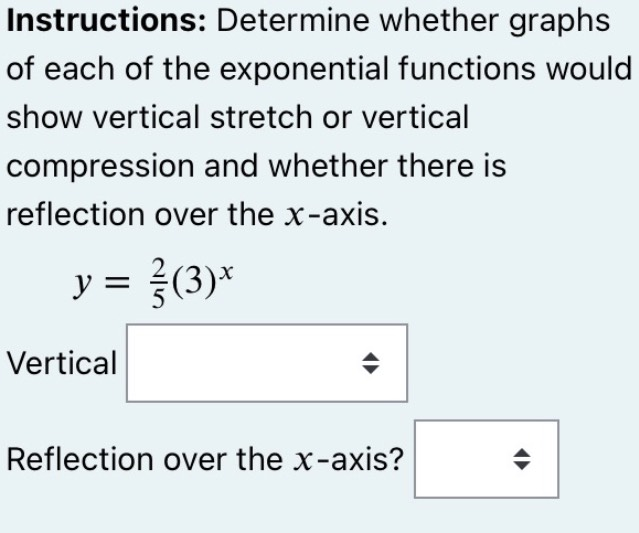### Still have math questions?

Algebra
QuestionInstructions: Determine whether graphs of each of the exponential functions would show vertical stretch or vertical compression and whether there is reflection over the $$x$$ -axis.

$$y = \frac { 2 } { 5 } ( 3 ) ^ { x }$$

Vertical ____

Reflection over the $$x$$ -axis? ____